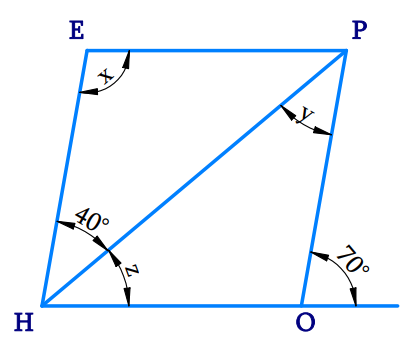# Ex.3.3 Q7 Understanding Quadrilaterals Solution-Ncert Maths Class 8

Go back to  'Ex.3.3'

## Question

The adjacent figure $$HOPE$$ is a parallelogram. Find the angle measures $$x, y$$ and $$z.$$ State the properties you use to find them.

Video Solution
Ex 3.3 | Question 7

## Text Solution

What is Known?

Given figure is a parallelogram.

What is Unknown?

Values of $$x, y, z.$$

Reasoning:

In a parallelogram, opposite angles are equal and adjacent angles are supplementary. Using this property, we can calculate the unknown angles.

Steps:Here,

\begin{align}\angle &{\rm{HOP }} + {\rm{ 7}}0^\circ = {\rm{ 18}}0^\circ {\rm{ }}\,\,\\&\left[ {{\text{Angles of linear pair}}} \right]\\\angle &{\rm{HOP}} = {\rm{18}}0^\circ - {\rm{7}}0^\circ \\\angle &{\rm{HOP}} = {\rm{11}}0^\circ \\\angle &{\rm{O }} = \angle E\left( {{\text{opposite angles are equal}}} \right)\\\therefore &{\rm{x}} = {\rm{11}}0^\circ {\rm{ }}\end{align}

\begin{align}\text{y}&={{40}^{\text{o}}}\\\text{ (Alternate }&\text{interior angles are equal) } \\\text{z}+{{40}^{\circ}}&={{70}^{\circ}}\\&\text{ (Corresponding angles) } \\ \text{z}&={{70}^{\circ}}-{{40}^{\circ}} \\ \text{z}&={{30}^{\circ}} \\ \end{align}

$\therefore {\text{ x }} = {\rm{ 11}}0^\circ ,{\text{ y }} = {\text{ 4}}0^\circ ,{\rm{ z }} = {\text{ 3}}0^\circ$

Learn from the best math teachers and top your exams

• Live one on one classroom and doubt clearing
• Practice worksheets in and after class for conceptual clarity
• Personalized curriculum to keep up with school